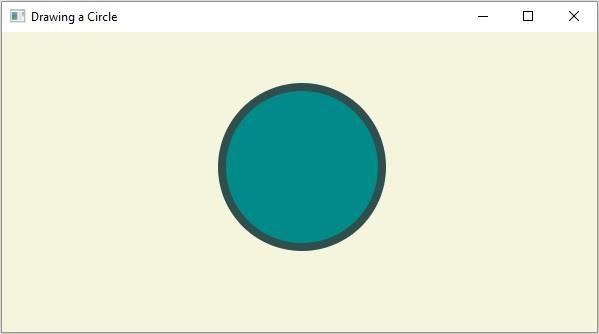# How to create a circle using JavaFX?

A circle is a line forming a closed loop, every point on which is a fixed distance from a center point. A circle is defined by its center and radius − distance from the center to any point on the circle.

In JavaFX a circle is represented by the javafx.scene.shape.Circle class. This class contains three properties they are −

• centerX − This property represents the x coordinate of the center of a circle, you can set the value to this property using the setCenterX() method.

• centerY − This property represents y coordinate of the center of a circle, you can set the value to this property using the setCenterY() method.

• radius − The radius of the circle in pixels, you can set the value to this property using the setRadius() method.

To create a circle you need to −

• Instantiate the class Circle.

• Set the required properties using the setter methods or, bypassing them as arguments to the constructor.

• Add the created node (shape) to the Group object.

## Example

import javafx.application.Application;
import javafx.scene.Group;
import javafx.scene.Scene;
import javafx.scene.paint.Color;
import javafx.stage.Stage;
import javafx.scene.shape.Circle;
public class DrawingCircle extends Application {
public void start(Stage stage) {
//Drawing a Circle
Circle circle = new Circle();
//Setting the properties of the circle
circle.setCenterX(300.0f);
circle.setCenterY(135.0f);
//Setting other properties
circle.setFill(Color.DARKCYAN);
circle.setStrokeWidth(8.0);
circle.setStroke(Color.DARKSLATEGREY);
//Setting the Scene
Group root = new Group(circle);
Scene scene = new Scene(root, 595, 300, Color.BEIGE);
stage.setTitle("Drawing a Circle");
stage.setScene(scene);
stage.show();
}
public static void main(String args[]){
launch(args);
}
}

## Output